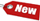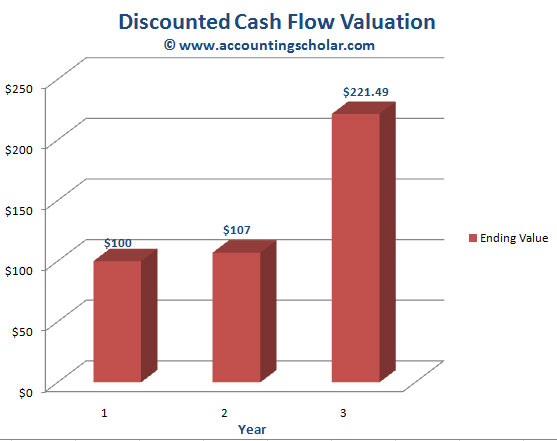Browse Accounting Lessons HereAccounting Terms & DefinitionsAccounting for Merchandising ActivitiesDebits and Credits (Double Entry Accounting)Business Valuation FormulasTime Value of Money & Present/Future ValuesComplex Debt & Equity InstrumentsCommon Stock & Shareholder's EquityAccounting & Finance RatiosValuing Common StockCorporate Income TaxesLower of Cost or Market (LCM) & Inventory ValuationChart of Accounts & BookkeepingBonds Payable & Long Term LiabilitiesCapital AssetsGAAP, Accrual & Cash Accounting, Information Commodity, Internal Controls & MaterialityWhat category of browser are you on this website? Accounting student (homework help) Finance professor (university research) Accounting manager (at work) Other Explore Careers in Accounting and FinanceVisit our section on Careers in Accounting & Finance to explore vast opportunities in this industry.

Chapter 4.11® - Discounted Cash Flow Valuations - Future Value of Multiple Cash Flows & Designing the Cash Flows Timeline

So far in the time value of money chapter, we have focused solely on the future value of a lump sum payment or present value of a single cash flow expected to be generated in the future. However more often than not, these valuations include multiple cash flow payments in the future. How do we account for those? For instance, if McDonalds is planning to open a new store, there will probably be a large cash outflow (investment) now and lots of cash inflows for many years in the future. In this chapter, we begin to explore investments of this nature.

Future Value of Multiple Cash Flows

Imagine you deposit \$100 today in an interest bearing account paying 7%. In one year, you will contribute an additional \$100. How much will you have in two years? This problem is easy to solve; at the end of the first year, you will have \$107 plus the second \$100 you deposit for a total of \$207. Then, you will leave this \$207 on deposit at 7% for the 2nd year. At the end of the 2nd year, this money will be worth:

2nd Year Worth = \$207 x 1.07 = \$221.49

1) Cash Flows Timeline

 0_________________ \$100 1____________________ \$100 2_____________ \$0 > (Time in years) > Cash Flows

2) Calculating the Future Value

 0_________________ \$100 1_____________________ \$100 2_____________ \$0 > (Time in years) > Cash Flows |____x1.07_________ +\$107 \$207___x1.07__________> \$221.49

The table above is a financial time line that literally draws the process of calculating future value of these two \$100 deposits in a format that is understandable by anyone. Drawing diagrams like this for solving complicated cash flows & future cash flow questions can be very helpful.

Here is how the set of calculations look like:

 Year 1 Investment = \$100 Interest earned = \$100 x 1.07 = \$107 Year 2 Investment = \$100 Total beginning balance at Year 2 = \$107 + \$100 Ending balance at Year 2 = \$207 x 1.07 = \$221.49

Here is how this data looks in graphical format:This graph above shows the growth of \$100 in year 1 to \$221.49 in 2 years after making another \$100 deposit in year 2, and earning an annual rate of return of 7% compounded annually.

From the diagrams, the first cash flow occurs today, which we have labelled as Time 0. This is why we have put \$100 as the first contribution under Column 0 because that’s when it was deposited. To make things easy for you, always start financial time lines with a 0 and NOT 1. The second cash flow occurs in one year from today, so we wrote this down at the point labelled Time 1. At the end of Year 2, we receive interest on our compounded or accumulated balance of \$207 and the calculation is:

 2nd Year Worth = \$207 x 1.07 = \$221.49 Thus, \$221.49 is the ending balance of year 2, or beginning balance of Year 3.

We could have made these calculations a little easier because when we calculated the future value of the two \$100 deposits, we simply calculated the balance as of the beginning of each year, then rolled that amount forward to the next year. The quicker way to do this is, the first \$100 was on deposit for 2 years, at a deposit rate of 7%, thus its future value is calculated as (using the future value discount factor):

 Future value factor = PV x (1 + r)t \$100 x 1.072 \$100 x 1.1449 = \$114.49

The second \$100 is on deposit for 1 year at 7%, thus its future value is:

 \$100 x 1.07 = \$107

The total future value is equal to the sum of these two future value calculations: \$114.49 + \$107 = \$221.49

Thus, from this example, there are two ways to calculate future values for multiple cash flows:

 1) Compound the accumulated balance forward one year at a time or 2) Calculate the future value of each cash flow first, and then add them up.